# Disdyakis dodecahedron

Disdyakis dodecahedron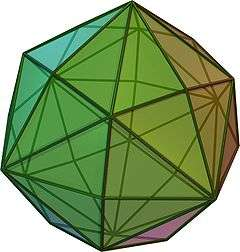Click on picture for large version

Spinning version

TypeCatalan solid
Conway notationmC
Coxeter diagramFace polygon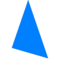scalene triangle
Faces48
Edges72
Vertices26 = 6 + 8 + 12
Face configurationV4.6.8
Symmetry groupOh, B3, [4,3], *432
Dihedral angle155° 4' 56"Dual polyhedrontruncated cuboctahedron
Propertiesconvex, face-transitive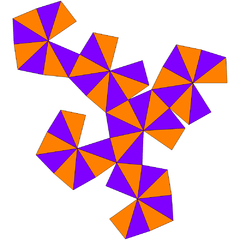Net

In geometry, a disdyakis dodecahedron, or hexakis octahedron or kisrhombic dodecahedron), is a Catalan solid with 48 faces and the dual to the Archimedean truncated cuboctahedron. As such it is face-transitive but with irregular face polygons. It superficially resembles an inflated rhombic dodecahedron—if one replaces each face of the rhombic dodecahedron with a single vertex and four triangles in a regular fashion one ends up with a disdyakis dodecahedron. More formally, the disdyakis dodecahedron is the Kleetope of the rhombic dodecahedron.

## Symmetry

It has Oh octahedral symmetry. Its collective edges represent the reflection planes of the symmetry. It can also be seen in the corner and mid-edge triangulation of the regular cube and octahedron, and rhombic dodecahedron.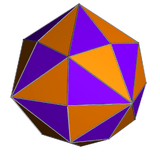Disdyakisdodecahedron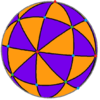Spherical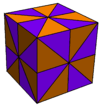Cubic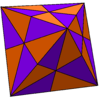Octahedral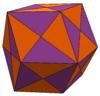Rhombicdodecahedral

Seen in stereographic projection the edges of the disdyakis dodecahedron form 9 circles (or centrally radial lines) in the plane. The 9 circles can be divided into two groups of 3 and 6 (drawn in purple and red), representing in two orthogonal subgroups: [2,2], and [3,3]:

Orthogonal Stereographic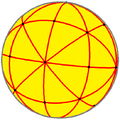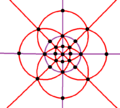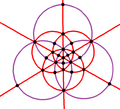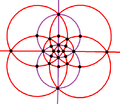## Dimensions

If its smallest edges have length 1, its surface area isand its volume is.

## Orthogonal projections

The truncated cuboctahedron and its dual, the disdyakis dodecahedron can be drawn in a number of symmetric orthogonal projective orientations. Between a polyhedron and its dual, vertices and faces are swapped in positions, and edges are perpendicular.

 Projectivesymmetry Image Dualimage       +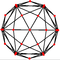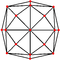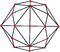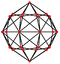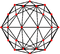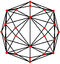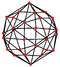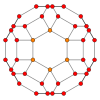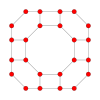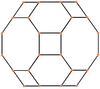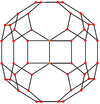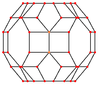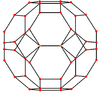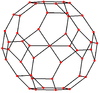## Related polyhedra and tilings

The disdyakis dodecahedron is one of a family of duals to the uniform polyhedra related to the cube and regular octahedron.

It is a polyhedra in a sequence defined by the face configuration V4.6.2n. This group is special for having all even number of edges per vertex and form bisecting planes through the polyhedra and infinite lines in the plane, and continuing into the hyperbolic plane for anyWith an even number of faces at every vertex, these polyhedra and tilings can be shown by alternating two colors so all adjacent faces have different colors.

Each face on these domains also corresponds to the fundamental domain of a symmetry group with order 2,3,n mirrors at each triangle face vertex.